### Home > CAAC > Chapter 9 > Lesson 9.3.1 > Problem9-64

9-64.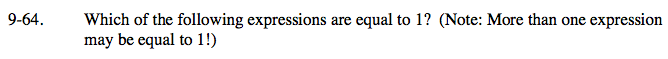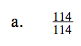Any number or expression divided by itself is equal to one.

Is the bottom of the fraction the same as the top?

The expression is equal to 1.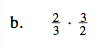Multiply the fraction.

$\frac{2}{3} \left( \frac{3}{2} \right) = \frac{6}{6}$

Follow the steps in part (a).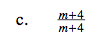Follow the steps in part (a).

The expression is equal to 1.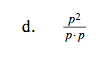Follow the steps in part (a).